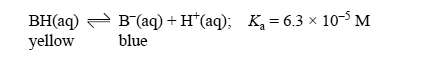Acid-base equilibrium 2007 VCE Bromophenol blue is a weak acid (represented as BH) that acts as an acid-base indicator. In solution the following equilibrium is established.At low pH bromophenol blue exists mainly as the acid, BH, which is yellow in colour, while at high pH it exists mainly as its conjugate base, B, which is blue. An intermediate colour is observed when the concentration of the acid and the concentration of the conjugate base are similar. Write an expression for Ka in terms of the concentrations of BH, B and H+. Solution When [BH] = [B-], the mixture appears green. Calculate the pH at which [BH] = [B-]. Solution Calculate the ratio [B-]/[BH] when the pH of a solution of bromophenol blue is 7. Solution What colour will the indicator solution appear at pH 7? Justify your answer SolutionOne litre of an aqueous solution of potassium hydroxide (KOH) has a pH of 12.0 at 25°C. The amount of solid KOH, in mol, that must be added to the solution to raise the pH to 13.0 would be A. 10-13 B. 10-12 C. 0.09 D. 0.10 Solution The amount of pure HCl gas, in mol, that must be added to the solution to lower the pH from pH 12.0 to 2.0 would be A. 10 B. 2.0 C. 0.02 D. 0.01 Solution